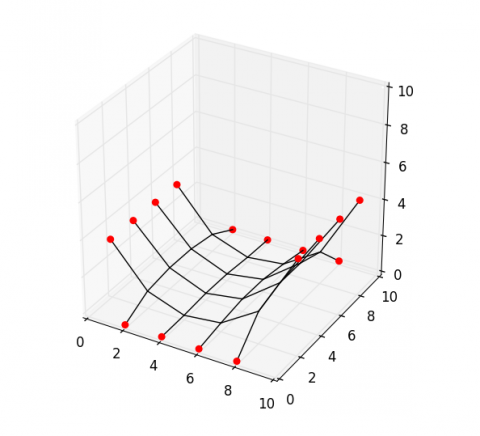## The force density method

In previous posts, we described how to read network data from a file and convert it into matrices relevant for structural calculations. Here, we will use the force density method to compute an equilibrium shape of the provided network data.

from numpy import array, zeros, float64
from scipy.sparse import coo_matrix, diags
from scipy.linalg import cho_solve, cho_factor

# Input data:
# -----------
# vertices: a list of unique xyz coordinates
# edges: a list of pairs of vertex indices
# points: index list of fixed vertices

m = len(edges)
n = len(vertices)

fixed = points
free  = list(set(range(n)) - set(fixed))

data = array([-1]*m + *m)
rows = array(range(m) + range(m))
cols = array(edges).reshape(2*m, order='F')
C    = coo_matrix((data, (rows, cols))).tocsr()
Ci   = C[:, free]
Cit  = Ci.transpose()
Cf   = C[:, fixed]
xyz  = array(vertices)
p    = zeros((n, 3), dtype=float64)
q    = array([1.0 for i in range(m)]).reshape((-1, 1))
Q    = diags([q.flatten()], )

A = Cit.dot(Q).dot(Ci)
b = p[free] - Cit.dot(Q.dot(Cf.dot(xyz[fixed])))

xyz[free] = cho_solve(cho_factor(A), b)


With this file (‘http://block.arch.ethz.ch/labs/samples/saddle.obj’) as input, you should get the following figure of equilibrium. For details on plotting network data, read this post.With the coefficient matrix A pre-factorized, we can easily compute different figures for different loading cases and/or boundary conditions.

Afactor = cho_factor(A.toarray())

p[free, 2] = -1.0
b          = p[free] - Cit.dot(Q.dot(Cf.dot(xyz[fixed])))
xyz[free]  = cho_solve(Afactor, b)